“Hide-and-Seek: Forcing a Network to be Meticulous for Weakly-supervised Object and Action Localization” proposed a weakly-supervised framework to improve object localization in images and action localization in videos. It was presented in International Conference on Computer Vision (ICCV) 2017 by Krishna Kumar Singh and Yong Jae Lee.

# Summary

• Problem Statement
• Most existing weakly-supervised methods localize only the most discriminative parts of an object rather than all relevant parts.
• This leads to suboptimal performance.
• Research Objective
• To improve object localization in images and action localization in videos.
• Solution Proposed: Hide-and-Seek
• The key idea is to hide patches in a training image randomly, forcing the network to seek other relevant parts when the most discriminative part is hidden.
• Contribution
• Introduce the idea of Hide-and-Seek for weakly-supervised localization and produce state-of-the-art object localization results on the ILSVRC dataset
• Demonstrate the generalizability of the approach on different networks and layers
• Extend the idea to the relatively unexplored task of weakly-supervised temporal action localization

# Introduction

• Previous weakly-supervised methods often fail to identify the entire extent of the object and instead localize only the most discriminative part

• Main Idea

• Proposed method make changes to the input image.
• The key idea is to hide patches from an image during training so that the model needs to seek the relevant object parts from what remains.
• By randomly hiding different patches in each training epoch, the model sees different parts of the image and is forced to focus on multiple relevant parts of the object beyond just the most discriminative one.
• This random hiding of patches will be only applied on training, not on testing.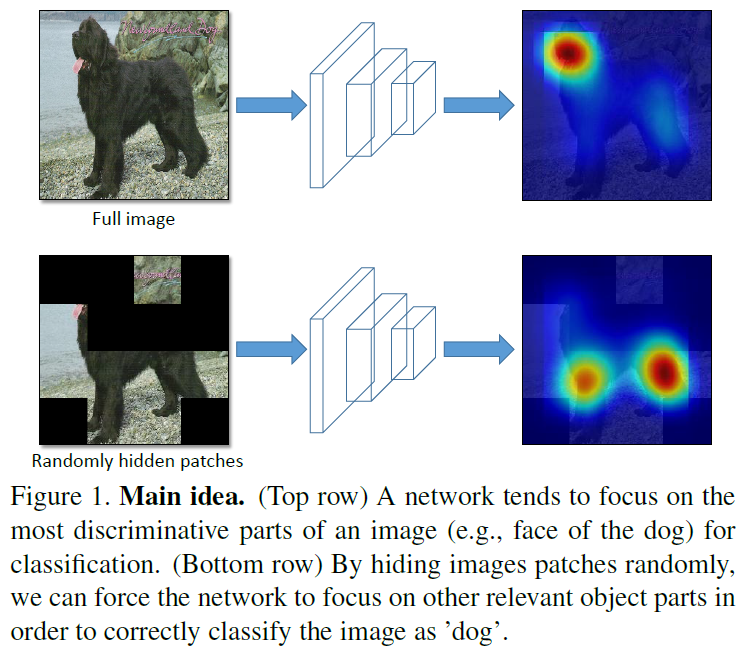# Proposed Method

## Weakly-supervised Object Localization

• Goal: To learn an object localizer that can predict both the category label as well as the bounding box for the object-of-interest in a new test image $I_test$
• Given a set of images $I_{set}={I_1, I_2, ..., I_N}$ where each image $I$ is labeled only with its category label.
• In order to learn the object localizer, the authors train a CNN which simultaneously learns to localize the object while performing the image classification task.
• Existing localizing method only focus on discriminative object parts, since they are sufficient for optimizing the classification task.
• In order to make the network to learn all of the relevant parts of an object, HaS randomly hide patches of each input image $I$ during training.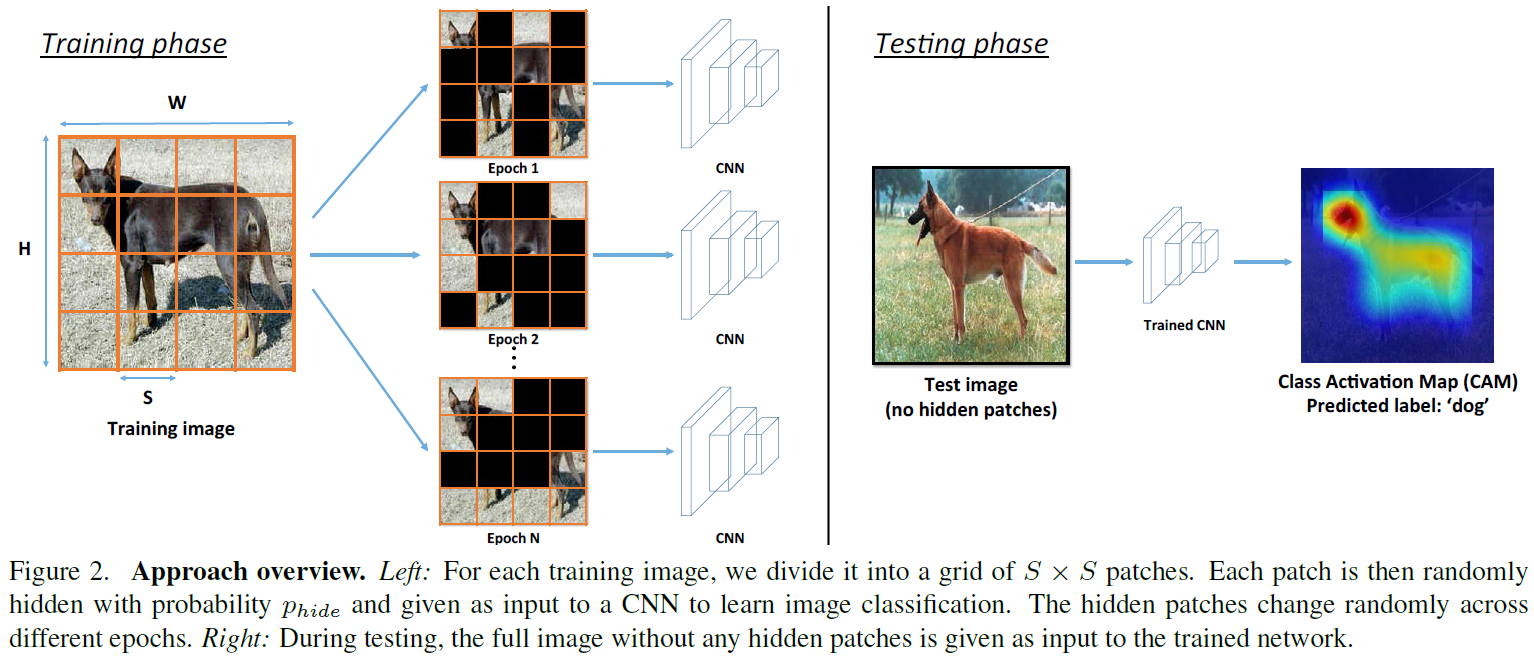### Hiding Random Image Patches

By hiding patches randomly, we can ensure the most discriminative parts of an object are not always visible to the network, and thus force it to also focus on other relevant parts of the object.

• Training Process of Hiding Ramdom Image Patches
1. With training image $I$ of size $W \times H \times 3$, we first divide it into a grid with a fixed patch size of $S \times S \times 3$.
2. It results in a total of $(W \times H)/(S \times S)$ patches.
3. Hide each patch with $p_{hide}$ probability.
4. Take the new image $I'$ with the hidden patches, and feed it as a training input to a CNN for classification.
• During testing, the full image without any patches hidden is given as input to the network.
• Previous study [L Bazzani et al., 2016] hides patches during testing.
• However, it has no significant effect on localization performance since the network has already learned to focus on the most discriminative parts during training.

### Setting the Hidden Pixel Values

Due to the discrepancy of hiding patches during training while not hiding patches during testing, the first convolutional layer activations during training versus testing will have different distributions. For a trained network to generalize well to new test data, the activation distributions should be roughly equal.

• Suppose we have a convolution filter $F$ with kernel size $K \times K$
• Three-dimensional weights $W = {w_1, w_2, ..., w_{k \times k}}$, which is applied to an RGB patch $X={x_1, x_2, ..., x_{k \times k}}$ in image $I'$.
• Denote $v$ as the vector representing the RGB value of every hidden pixel.
• There are three types of activations as shown in the below figure.
1. $F$ is completely within a visible patch
2. $F$ is completely within a hidden patch
3. $F$ is partially within a hidden patch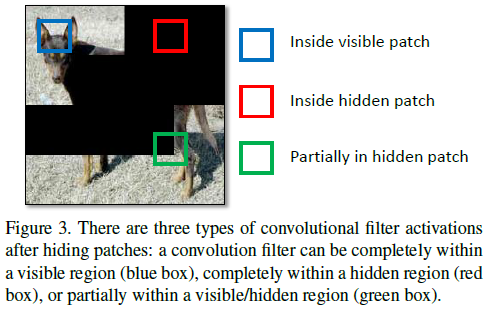• How to resolve the issue
• Set the RGB value $v$ of a hidden pixel to be equal to the mean RGB vector of the images over the entire dataset.
• Why would this work?
• Essentially, the authors are assuming that in expectation, the output of a patch will be equal to that of an average-valued patch.
• The outputs of both the second and third cases will be matched with the expected output during testing (i.e., of a fully-visible patch).
• This process is related to the scaling procedure in dropout.
• Empirically, the authors find that setting the hidden pixel in this way is crucial for the network to behave similarly during training and testing.

### Object Localization Network Architecture

HaS approach of hiding patches is independent of the network architecture and can be used with any CNN designed for object localization.

• For experiments, the authors use the network of [Zhou et al.,], which performs global average pooling (GAP) over the convolution feature maps to generate a class activation map (CAM) for the input image that represents the discriminative regions for a given class.
• To generate a CAM for an image, global average pooling is performed after the last convolutional layer and the result is given to a classification layer to predict the image’s class probabilities.
• The weights associated with a class in the classification layer represent the importance of the last convolutional layers feature maps for that class.
• Denote $F={F_1, F_2, ..., F_M}$ to be the $M$ feature maps of the last convolutional layer and $W$ as the $N \times M$ weight matrix of the classification layer, where $N$ is number of classes.
• The CAM for class $c$ for image $I$ is:
• Given the CAM for an image, we first threshold the CAM to produce a binary foreground/background map, and then find connected components among the foreground pixels.
• Finally, we fit a tight bounding box to the largest connected component.

## Weakly-supervised Action Localization

• Goal: Given a set of untrimmed videos $V_{set} = {V_1, V_2, ..., V_N}$ and video class labels, the goal is to learn an action localizer that can predict the label of an action as well as its start and end time for a test video $V_{test}$.

• Problem: The key issue is that, a network focus mostly on the highly-discriminative frames in order to optimize classification accuracy instead of identifying all relevant frames.

• Solution: Hide frames in videos to improve action localization

• First, uniformly sample video $F_{total}$ frames from each videos.
• Second, divide the $F_{total}$ frames into continuous segments of fixed size $F_{segment}$.
• Third, hide each segment with probability $p_{hide}$ before feeding it into a deep action localizer network.
• Applied thresholding on the map to obtain the start and end times for the action class.

# Experiments

## Datasets and Evaluation Metrics

• Experiment of Object Localization
• Used ILSVRC 2016 dataset
• Used three evaluation metrics to measure performance:
1. Top-1 localization accuracy (Top-1 Loc): fraction of images for which the predicted class with the highest probability is the same as the ground-truth class and the predicted bounding box for the class has more than 50% Intersection over Union (IoU) with the ground-truth box.
2. Localization accuracy with known ground-truth class (GT-known Loc): fraction of images for which the predicted bounding box for the ground-truth class has more than 50% IoU with the ground-truth box.
3. Classification accuracy (Top-1 Clas): measure the impact of Hide-and-Seek on image classification performance
• Experiemnt of Action Localization
• Used THUMOS 2014 dataset
• For evaluation, computed mean average precision (mAP), and consider a prediction to be correct if it has IoU > $\theta$ with ground-truth.

## Implementation Details

• Models for Object Localization
• For both AlexNet-GAP and GoogLeNet-GAP, the output of the last conv layer goes to a global average pooling (GAP) layer, followed by a softmax layer for classification.
• For action localization, the authors compute C3D fc7 features using a model pre-trained on Sports 1 million.

## Experimental Results

Object Localization Quantitative Results

• Localization accuracy on ILSVRC validation data with different patch sizes (16, 32, 44, 56) for hiding.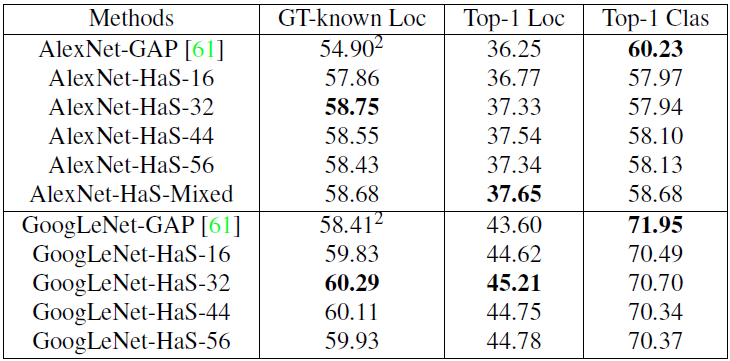• Localization accuracy on ILSVRC validation data compared to state-of-the-art.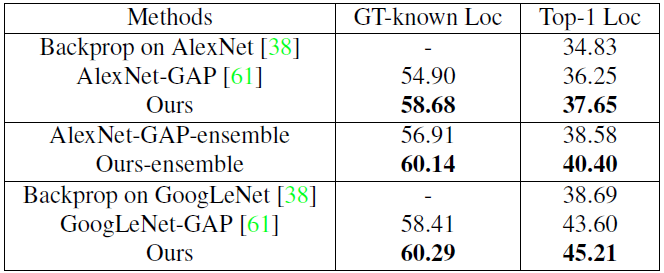Object Localization Qualitative Results

• Compared Hide-and-Seek approach with AlexNet-GAP on the ILVRC validation data.
• Hide-and-Seek approach localizes multiple relevant parts of an object whereas AlexNet-GAP mainly focuses only on the most discriminative parts.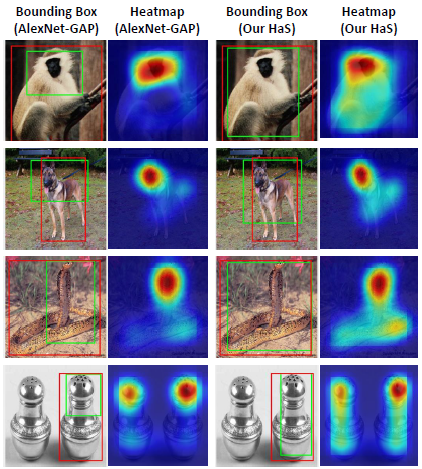Further Analysis of Hide-and-Seek

• Comparison with dropout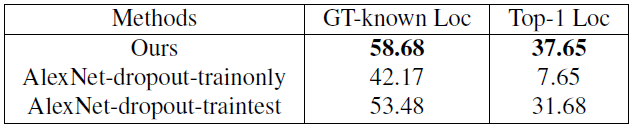• Global average pooling (GAP) vs. global max pooling (GMP)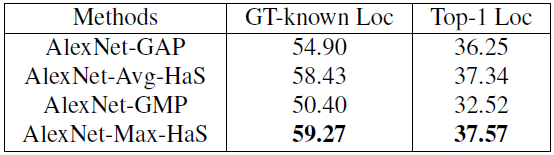• Applying Hide-and-Seek to the first conv layer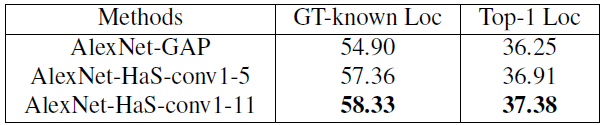• Varying the hiding probability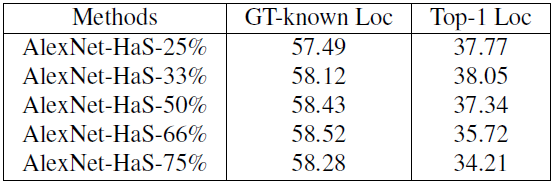• Action localization accuracy on THUMOS validation data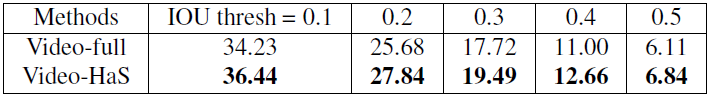• Paper: Hide-and-Seek: Forcing a Network to be Meticulous for Weakly-supervised Object and Action Localization [Link]
• Github: Hide-and-Seek (Implementation by the paper author) [Link]